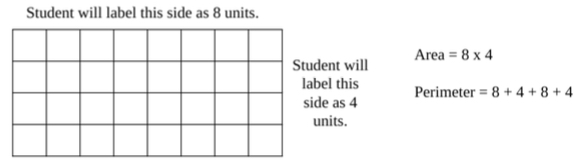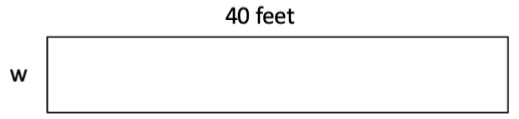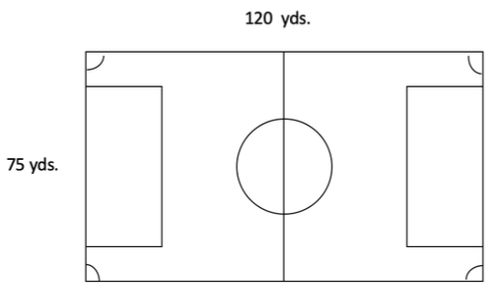# MA.4.GR.2.1Export Print
Solve perimeter and area mathematical and real-world problems, including problems with unknown sides, for rectangles with whole-number side lengths.

### Clarifications

Clarification 1: Instruction extends the development of algebraic thinking where the symbolic representation of the unknown uses a letter.

Clarification 2: Problems involving multiplication are limited to products of up to 3 digits by 2 digits. Problems involving division are limited to up to 4 digits divided by 1 digit.

Clarification 3: Responses include the appropriate units in word form.

General Information
Subject Area: Mathematics (B.E.S.T.)
Strand: Geometric Reasoning
Status: State Board Approved

## Benchmark Instructional Guide

• Perimeter

### Vertical Alignment

Previous Benchmarks

Next Benchmarks

### Purpose and Instructional Strategies

The purpose of this benchmark is for students to connect perimeter and area problems to algebraic concepts to find the measures of unknown side lengths. This new idea builds from solving area and perimeter problems with whole number side lengths when using models and formulas in grade 3 (MA.3.GR.2.3) and will form the foundation for problems involving fractional and decimal side lengths in grade 5 (MA.5.GR.2.1).
• During instruction, students should use a letter (variable) to represent the missing side length and have experiences solving for unknowns in perimeter situations with a given area and vice-versa.
• Instruction includes having students use the fact that opposite sides in rectangles and squares are equal when solving problems involving area and perimeter.

### Common Misconceptions or Errors

• Students frequently confuse area and perimeter. Instruction should provide lots of opportunity for students to work with both measures on the same object and have them explain which measure is area and which is perimeter and why? Instruction should also focus on naming the units properly.

### Strategies to Support Tiered Instruction

• Instruction provides many opportunities for students to work with both measures on the same object and explain which measure is area and which is perimeter and why. Instruction should also focus on naming the units properly
• Instruction includes finding both the area and perimeter in real world examples and having students explain how they solved for both.
• For example, when provided with examples like the following, students use the measurements provided to create an equation to find area and perimeter and explain the difference. “A rectangular garden is being built at the school. The dimensions for the garden are 8 feet by 4 feet. Write and solve an equation to find the area of the garden and an equation to find the perimeter of the garden.”
• The teacher provides students with images created using square tiles. Student count and labels the side lengths based on the tiles, then write equations to show how they would find the area and how they would find the perimeter.
• For example: When provided with an image like the one shown below, students label each side length based on the number of tiles and write an equation for perimeter and then count the units around the outside of the figure to confirm their solution. Students multiply the length and width to find area and then count the number of squares that make up the figure to confirm their solution.The perimeter of the patio below is 98 square feet.What is the area of the patio?

### Instructional Items

Instructional Item 1

A soccer field with its dimensions is shown.Which equation can be used to find the area of the soccer field?
• a. 75 yards + 120 yards = A yards
• b. 75 yards + 75 yards + 120 yards + 120 yards = A yards
• c. 75 yards× 120 yards = A square yards
• d. 75 yards × 120 yards × 75 yards × 120 yards = A square yards

*The strategies, tasks and items included in the B1G-M are examples and should not be considered comprehensive.

## Related Courses

This benchmark is part of these courses.
5012060: Mathematics - Grade Four (Specifically in versions: 2014 - 2015, 2015 - 2022, 2022 and beyond (current))
7712050: Access Mathematics Grade 4 (Specifically in versions: 2014 - 2015, 2015 - 2018, 2018 - 2022, 2022 and beyond (current))
5012055: Grade 3 Accelerated Mathematics (Specifically in versions: 2019 - 2022, 2022 and beyond (current))
5012015: Foundational Skills in Mathematics 3-5 (Specifically in versions: 2019 - 2022, 2022 and beyond (current))

## Related Access Points

Alternate version of this benchmark for students with significant cognitive disabilities.
MA.4.GR.2.AP.1: Solve perimeter and area mathematical and real-world problems for rectangles with given whole-number side lengths.

## Related Resources

Vetted resources educators can use to teach the concepts and skills in this benchmark.

## Formative Assessments

What Is the Perimeter of the Lettuce Section?:

Students are asked to use known lengths, areas, and perimeters to determine the perimeter of one section of a rectangle.

Type: Formative Assessment

Using Area and Perimeter:

Students are asked to solve real world problems by applying the formulas for area and perimeter.

Type: Formative Assessment

Fencing a Garden:

Students are given the area and width of a rectangle in the context of a word problem and are asked to determine the perimeter.

Type: Formative Assessment

Applying Area and Perimeter:

Students are asked to find the dimensions of rectangles by applying the formulas for area and perimeter.

Type: Formative Assessment

Finding the Perimeter of a Polygon with Missing Sides:

Students are asked to find the perimeter of a hexagon in which the lengths of two sides are not given but can be found.

Type: Formative Assessment

What Is the Missing Length?:

Students are asked to find the length of a missing side on two polygons given the perimeter of each and the lengths of the other sides.

Type: Formative Assessment

## Lesson Plans

Volunteer Trash Cleanup:

In this Model Eliciting Activity (MEA), students will help a volunteer coordinator choose cleanup projects that will have the greatest positive impact on the environment. Students will learn about how litter and pollution can affect wildlife as well as how cleanup efforts can help. They will discuss the importance of volunteering in the community and utilize math skills such as calculating area in deciding how to rank the different cleanup projects.

Type: Lesson Plan

New Puppy's Pen:

The purpose of this lesson is to help students find the missing side's length for rectangular area problems, when the total area and one side's length is given. The use of square tiles, then graph paper and equations are used throughout the lesson to help students progress from conceptual to procedural knowledge.

Type: Lesson Plan

Its All Around But Covered Up:

Students explore missing dimension challenges in real world area situations.

Type: Lesson Plan

Draw a blueprint of your dream house floor plan.:

This lesson will help your students learn about area and perimeter while imagining and drawing a blueprint of their dream house floor plan. They will have so much fun drawing and creating their blueprint they will forget that they are actually learning how to find area and perimeter.

Type: Lesson Plan

Park Planning:

Students are asked to plan a playground for a new park within a given budget and area limit. They will analyze the best use of playground equipment using a data table of area requirements and cost. Students will convert units within a single measurement system, calculate the area of a rectangle, and perform addition/subtraction calculations involving money using decimal notation.

Type: Lesson Plan

Pioneer Places:

In this MEA, students decide which type of pioneer dwelling was the best to build if you were traveling west.

Type: Lesson Plan

Same Perimeter, Different Area:

In this lesson, students are presented with a problem that requires them to create rectangles with the same perimeter but different areas.  Students also search for relationships among the perimeters and areas of different rectangles and find which characteristics produce a rectangle with the greatest area.

Type: Lesson Plan

Bre and Brent the Builders:

The students will be able to use prior knowledge of the concept of area to relate area to multiplication and discover the formula: base x height. Students will practice by composing two-dimensional squares and rectangles and apply the area formula to build a birdhouse.

Type: Lesson Plan

Wallpaper Woes Money Math: Lessons for Life:

Students hear a story about a middle-school student who wants to redecorate his bedroom. They measure the classroom wall dimensions, draw a scale model, and incorporate measurements for windows and doors to determine the area that could be covered by wallpaper. Students then hear more about the student's redecorating adventure and learn about expenses, budget constraints, and tradeoffs.

Type: Lesson Plan

## Original Student Tutorials

Dream House Dilemma, Part 2: Perimeter:

Learn to calculate the perimeter of rectangular and composite shapes to help April finish designing her dream home in this interactive tutorial.

This is the second in a three-part series. Click below to open the other tutorials in the series.

Type: Original Student Tutorial

Dream House Dilemma Part 3: Perimeter and a Missing Side:

Learn how to calculate perimeter and find a missing side measurement for a shape given the perimeter in this interactive tutorial.

This is the third in a three-part series about designing a dream house.  Click below to open the other tutorials in this series.

Type: Original Student Tutorial

Dream House Dilemma Part 1: Area:

Help April calculate area and missing measurements for items in her perfect dream home in this interactive tutorial.

This is the first in a three-part series.  Click below to open the other tutorials in this series

Type: Original Student Tutorial

Karl's Garden:

The purpose of the task is for students to solve a multi-step multiplication problem in a context that involves area. In addition, the numbers were chosen to determine if students have a common misconception related to multiplication. Since addition is both commutative and associative, we can reorder or regroup addends any way we like. Students often believe the same is true for multiplication.

## Tutorials

Area and Perimeter Word Problem: Table Dimensions:

This Khan Academy tutorial video presents a step-by-step solution for finding the length and width of a table when given its area and perimeter.

Type: Tutorial

Comparing Areas of Plots of Land:

Find area of two rectangles to solve a word problem.

Type: Tutorial

Comparing areas and perimeters of rectangles:

In this tutorial video from Khan Academy, explore the relationship between area and perimeter. For example, if you know the area and the length, can you find the perimeter?

Type: Tutorial

## STEM Lessons - Model Eliciting Activity

Park Planning:

Students are asked to plan a playground for a new park within a given budget and area limit. They will analyze the best use of playground equipment using a data table of area requirements and cost. Students will convert units within a single measurement system, calculate the area of a rectangle, and perform addition/subtraction calculations involving money using decimal notation.

Pioneer Places:

In this MEA, students decide which type of pioneer dwelling was the best to build if you were traveling west.

## MFAS Formative Assessments

Applying Area and Perimeter:

Students are asked to find the dimensions of rectangles by applying the formulas for area and perimeter.

Fencing a Garden:

Students are given the area and width of a rectangle in the context of a word problem and are asked to determine the perimeter.

Finding the Perimeter of a Polygon with Missing Sides:

Students are asked to find the perimeter of a hexagon in which the lengths of two sides are not given but can be found.

Using Area and Perimeter:

Students are asked to solve real world problems by applying the formulas for area and perimeter.

What Is the Missing Length?:

Students are asked to find the length of a missing side on two polygons given the perimeter of each and the lengths of the other sides.

What Is the Perimeter of the Lettuce Section?:

Students are asked to use known lengths, areas, and perimeters to determine the perimeter of one section of a rectangle.

## Original Student Tutorials Mathematics - Grades K-5

Dream House Dilemma Part 1: Area:

Help April calculate area and missing measurements for items in her perfect dream home in this interactive tutorial.

This is the first in a three-part series.  Click below to open the other tutorials in this series

Dream House Dilemma Part 3: Perimeter and a Missing Side:

Learn how to calculate perimeter and find a missing side measurement for a shape given the perimeter in this interactive tutorial.

This is the third in a three-part series about designing a dream house.  Click below to open the other tutorials in this series.

Dream House Dilemma, Part 2: Perimeter:

Learn to calculate the perimeter of rectangular and composite shapes to help April finish designing her dream home in this interactive tutorial.

This is the second in a three-part series. Click below to open the other tutorials in the series.

## Student Resources

Vetted resources students can use to learn the concepts and skills in this benchmark.

## Original Student Tutorials

Dream House Dilemma, Part 2: Perimeter:

Learn to calculate the perimeter of rectangular and composite shapes to help April finish designing her dream home in this interactive tutorial.

This is the second in a three-part series. Click below to open the other tutorials in the series.

Type: Original Student Tutorial

Dream House Dilemma Part 3: Perimeter and a Missing Side:

Learn how to calculate perimeter and find a missing side measurement for a shape given the perimeter in this interactive tutorial.

This is the third in a three-part series about designing a dream house.  Click below to open the other tutorials in this series.

Type: Original Student Tutorial

Dream House Dilemma Part 1: Area:

Help April calculate area and missing measurements for items in her perfect dream home in this interactive tutorial.

This is the first in a three-part series.  Click below to open the other tutorials in this series

Type: Original Student Tutorial

Karl's Garden:

The purpose of the task is for students to solve a multi-step multiplication problem in a context that involves area. In addition, the numbers were chosen to determine if students have a common misconception related to multiplication. Since addition is both commutative and associative, we can reorder or regroup addends any way we like. Students often believe the same is true for multiplication.

## Tutorials

Area and Perimeter Word Problem: Table Dimensions:

This Khan Academy tutorial video presents a step-by-step solution for finding the length and width of a table when given its area and perimeter.

Type: Tutorial

Comparing Areas of Plots of Land:

Find area of two rectangles to solve a word problem.

Type: Tutorial

Comparing areas and perimeters of rectangles:

In this tutorial video from Khan Academy, explore the relationship between area and perimeter. For example, if you know the area and the length, can you find the perimeter?

Type: Tutorial

## Parent Resources

Vetted resources caregivers can use to help students learn the concepts and skills in this benchmark.

Karl's Garden:

The purpose of the task is for students to solve a multi-step multiplication problem in a context that involves area. In addition, the numbers were chosen to determine if students have a common misconception related to multiplication. Since addition is both commutative and associative, we can reorder or regroup addends any way we like. Students often believe the same is true for multiplication.